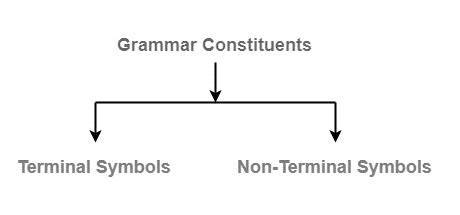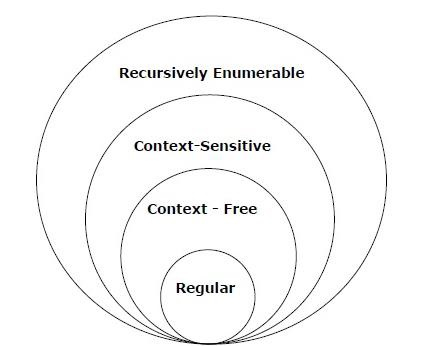# Explain the concept of grammar in TOC

Data Structure AlgorithmsComputer ScienceComputers

Grammar in theory of computation is a finite set of formal rules that are generating syntactically correct sentences.

The formal definition of grammar is that it is defined as four tuples −

G=(V,T,P,S)
• G is a grammar, which consists of a set of production rules. It is used to generate the strings of a language.

• T is the final set of terminal symbols. It is denoted by lower case letters.

• V is the final set of non-terminal symbols. It is denoted by capital letters.

• P is a set of production rules, which is used for replacing non-terminal symbols (on the left side of production) in a string with other terminals (on the right side of production).

• S is the start symbol used to derive the string.

Grammar is composed of two basic elementsTerminal Symbols - Terminal symbols are the components of the sentences that are generated using grammar and are denoted using small case letters like a, b, c etc.

Non-Terminal Symbols - Non-Terminal Symbols take part in the generation of the sentence but are not the component of the sentence. These types of symbols are also called Auxiliary Symbols and Variables. They are represented using a capital letter like A, B, C, etc.

## Example 1

Consider a grammar

G = (V , T , P , S)

Where,

V = { S , A , B }    ⇒ Non-Terminal symbols
T = { a , b }        ⇒ Terminal symbols
Production rules    P = { S → ABa , A → BB , B → ab , AA → b }
S = { S }           ⇒ Start symbol

## Example 2

Consider a grammar

G=(V,T,P,S)

Where,

V= {S, A, B}    ⇒ non terminal symbols
T = { 0,1}      ⇒ terminal symbols
Production rules P = { S→A1B
A→0A| ε
B→0B| 1B| ε }
S= {S}          ⇒ start symbol.

## Types of grammar

The different types of grammar −

GrammarLanguageAutomataProduction rules
Type-0Recursively enumerableTuring machineNo restriction
Type-1Context-sensitiveLinear-bounded non-deterministic machineαAβ→αγβ
Type-2Context-freeNon-deterministic push down automataA→γ
Type-3RegularFinite state automataA→αB
A→α

The diagram representing the types of grammar in the theory of computation (TOC) is as follows −